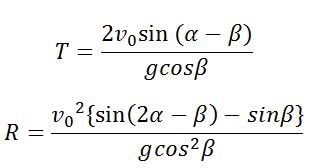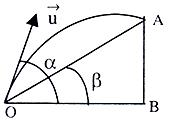# Parameters of a Projectile on an incline moving upwards Calculator

This CalcTown calculator calculates the parameters (i.e time of flight and range) of a Projectile projected upwards on an incline.

m/s

#### Result

s
mClick here to view image

where

α=Angle of Projection of the body w.r.t horizontal

β=Angle of the incline

v0=Projection velocity